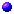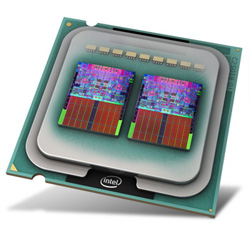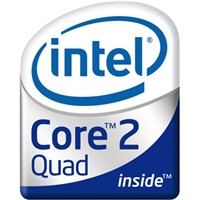# Parallel Systems - Practica

Until 2016, the practicum was part of the course. Now, we discuss some of the exercises during the theory.

###Utility classes (check main method for usage)v1.1 jar-filev1 jar-filecodeour default libraries (install at least mpj.jar)

• edu.cmu.tetrad.data.LongColumn to average out results of multiple experiments.
• be.ac.vub.ir.data.Chart to show data.
• be.ac.vub.ir.data.FrequencyTable to show a frequency table

###Room 4k228 computer specificationsCPU Speed: 2.66 GHz 64 bit processor Level 2 cache: 2 x 4 MB (each L2 cache is shared between 2 cores) Level 1 instruction cache: 4 x 32 KB Level 1 data cache: 4 x 32 KB Architecture RAM: 4 GB Graphics Card: NVIDIA GeForce 9400GT has 16 'cores' and supports CUDA for general purpose computing...###Exercises

Tips:
• Use -Xmx256M as VM argument if more memory is needed (set in Eclipse for sequential or multithreaded algorithms or after the mpjrun.bat for message-passing algorithms)
• Call the garbage collector (between 2 experiments) with Runtime.getRuntime().gc();Exercises 1-10

 Memory Access Overhead (NOT THIS YEAR)
1.   Test the cache miss penalty with the array traveling program ArrayWalking.   Solution
• Can you estimate the cost of a Level 1 and Level 2 cache miss?
• Can you estimate the relative memory latency Lambda (PPP page 66) for the different memory levels?

2.   Test if latency hiding can be obtained with file reading from system and from url in a separate thread (FileReading.java).
• By running on multiple cores, by running on one core (set affinity of eclipse process to 1 core in Windows' Task Manager).
3.   Implement a multithreaded fibonacci algorithm (Fibonacci.java).
• Base the calculations on the following recursive definition. This is of course not the most intelligent solution, actually terribly dumb. Let's assume that it is a good way of calculating fibonacci. Since it generates a lot of computations, it is good for obtaining speedup when run in parallel!
• Fibonacci (n) = Fibonacci (n-1) + Fibonacci (n-1)  if n > 2
• Fibonacci (n) = 1  if n = 1 or n = 2
• Fibonacci (n) = 0  if n < 1
• There are several ways to decompose the problem. Try to find a solution for which you can parameterize the number of threads.
• Study the performance in function of the number of threads.
• Try to get an ideal speedup (= number of cores).
• If this ideal is not met, try to find an explanation!
• Advanced questions: Is it a load-balanced solution? Count the number of threads. Count the number of work of each thread or time the computation time of each thread.
4.   Implement a multithreaded matrix multiplication.
• Try to make a solution in which you can set the number of threads.
• What speedup do you obtain?
• What is the maximal number ot threads?
• How does the speedup evolves with increasing number of threads? And with increasing matrix size?

5.   Test the the performance of various Count3s implementations.
• The given first solution is wrong. Why?
• What happens if you limit the cores to 1 on which your program may run?
• Explore the following solutions (their correctness and their performance):
1. This is the given solution without any form of synchronization.
2. Try to protect the counter variable with a boolean preventing multiple access to the counter. Does this work?
• Use yield() to give hints to the OS to do a context switch.
3. Do the race conditions disappear by making the count variable volatile?
4. Use synchronized to protect the critical section. There are 2 ways to do this.
5. Use a private counter to increase the performance.
• Lin & Snyder (page 37) claim that padding increases performance (overcoming false sharing). Test the claim?
• Test whether false sharing happens when a (global) array is used in which each thread uses one element as private counter (idea of student Thierry Renaux, 2011). Try if padding helps to overcome false sharing.
• For an example of how padding might improve performance, examine the following code: False Sharing
(idea of student Cristoph de Troyer, 2014)
• Visualization of speedup on 8 cores
6. Solve Count3s by making use of an atomic counter (see java.util.concurrent.atomic package, Java API Doc).
7. Correct solution 2 by making use of AtomicBoolean (idea of student Sven Claeys, 2009).
8. Alternative for 5: let the main thread globalize the private counters (idea of student Robrecht Dewaele, 2010).
9. Can you invent another solution?

6.   Check Invariance-breaking with volatile (Slide 55). Solution
• In the threads, do a lot of updates of the upper and lower values to generate race conditions. Check the invariant each time, so that you see when it is broken.
• Don't put extra print statements in the threads. They will considerably slow down the threads and lower the chance of a race condition.
• Why don't you get a thread-safe solution with volatile? Which solution gives a correct program?
• Test it with at least 10.000.000 iterations.
• Can something go wrong with CheckInvariant?
7.   Break the 'monitorness' of an object so that two synchronized methods (here: f1 and f2) can be accessed simultaneously. Solution
• Verify first the monitorness of the ObjectAsMonitor by comparing synchronized with unsynchronized methods.
8.   Implement a Barrier (PPP page 193) and call the barrier in the previous exercise after each iteration. Solution
• Replaces the join in the previous exercise with a barrier call at the end of the thread and in the main.
• Optional: Implement a split-phase barrier (PPP page 193) and uses it in the previous example after each iteration. The condition is that a thread can only start the next iteration if everybody has finished f1(). f2() is therefore the useful work that can be done while waiting.
• As correctly pointed out by Christophe De Troyer, the solution is a bit more complex, especially if you are going to reuse the barrier object iteratively:
1. We should take into account the possibility if spurious wakeups
2. If the same barrier is used repetitively you should ensure that all threads have left the barrier before early threads already start entering.
Indeed, the java NotifyAll does not guarantee that the ownership is passed to the awakened threads: “after activation due to a notify, the inactive thread start wanting to run in the synchronized block again, but is treated equaly with all other threads that wants it”
Christophe De Troyer's November 2014 solution takes care of these issues!
9.   Implement a multithreaded solution for the following matrix update algorithm. See figure and Wavefront.java.
• The matrix is updated row-by-row from left to right. Each element is updated by adding the latest value of the upper element and by subtracting the left element.
• Implement a highly-parallel solution which is correct. Check the correctness of the parallel result. The performance is not the major goal here.
• The parallelism lies in the wavefront, as indicated on the figure.
• Hint: use one thread per row.
• How could you make it faster?
• Optional: do the update multiple times.
10. Implement a multithreaded solution for this iterative algorithm. Start from BarrierWithReduction.java. Solution
• The given program is a simple version of an iterative algorithm in which local computations have to be followed by a global calculation (reduction), as shown by this figure. This structure appears in several parallel algorithms, such as a parallel Dijkstra algorithm. The simple program is given so that you can concentrate on the synchronization, which is not trivial.
• Create a thread that calculates the value of each array element. Create a separate 'master' thread that does the reduction.
• Make sure it is 100 % thread-safe and all threads finish.
11.   An application of the double condition problem (cf PPP p. 173) with a deadlock: ApplesOrangesWithDeadlock.java. Make it deadlock-free.
• There are apple producer threads and there are orange producers. The first consume 1 apple and 1 orange to produce 2 apples, the second consume 1 apple and 1 orange to produce 2 oranges.
• Note that the code is written in Pthread-style as you would do in C. It uses Locks and Condition Variables.
• Note that if you remove the sleep() before the consume the application doesn't seem to deadlock.
12.   Computing the surface of the Mandelbrot set Mandelbrot.txt.
• You are given a template to compute the surface of a Mandelbrot set. Implement a multithreaded version of this code.
• The task here is to get as good a speedup as possible. Think how to decompose the problem to achieve this

OPTIONAL: Implement an efficient and deadlock-free solution for the Dining Philosophers problem.
• Efficiency is in terms of the time the philosophers are hungry. You should try to minimize starvation time.

 Message-passingExercises code 13-18  -  FastMPJ Download   -  MPJExpress Quick Start (Installation and user guide, Environment variables for FastMPJ , etc)  -   MPJ Express API doc  -  Quick reference 1 and page 2

13.   Implement a message-passing CountingPrimes algorithm (start from and  ).     MPITest
• What is the efficiency of the parallel solution? Does it maximally exploits the parallel processing power? Can you optimize the solution?
• Study the speedup of CountingPrimes in function of array size and number of processes.
• Compare it with the message-passing Counting3s solution. Which is more efficient?
• Study the message-passing Counting3s solution. Does it give a good speedup? Why (not)?
• If not done in your first solution, try to use MPI's collective communication operations in a second version.
14.   Study the default send & receive protocol (DefaultProtocol.java) which can result in a deadlock situation.
• MPJ Readme (check protocol switch limit)
• Implement at least 3 different solution that avoids the possibility of a deadlock.

15.   Implement a message-passing version of the BarrierWithReduction algorithm (exercise 10)

16.   Implement a message-passing version of the matrix update algorithm using the wavefront approach (exercise 9).    Complete solution

17.   Java provides automatic object serialization.
• Sun Documentation  and examples
• Object2ByteArray shows in what bytestream an object is transformed.
• Object2File shows object writing and reading to file.
• Try changing serialVersionUID.
• ObjectCommunication shows how the bytestream can be send through MPI.
• Optional: The previous solution can be optimized:
• By creating your own OutputStream. ByteArrayOutputStream.toByteArray() gives a copy of the byte array.
• The byte array of Object serialization can happen more dense by implementing the Externalizable interface.
• Now that we have seen how to serialize and communicate objects, it is clear that sending objects is possible in MPI.
18. NOT THIS YEAR. Test the communication performance of point-to-point communication.
• Measure the communication time/per integer for increasing message sizes, SendModes.java gives you the opportunity to do so.
• This code lets you test different send modes.
• Run with the -H options to see the program arguments.
• How much computations do I have to do to make it worth to do it remotely? The computation time should exceed the time to send the data. Measure the boundary value between speedup and slowdown.
• Measure a message-passing equivalent for Lambda (the relative memory latency).
• Also measure the performance characteristics for processes running on the same processor.

 GPU computing

 Performance Analysis (NOT THIS YEAR)

23.   Design and do a performance analysis of a parallel pipeline algorithm.
• This was an exam question in january 2008.
• Design (implementing is not necessary) a generic parallel solution for algorithms that can be pipelined. The problem class is defined as follows:
• We have nd data packages that have to be processed by a np process objects.
• Each process object has to process the data packages in the right order, because its state is changed after the processing of each data package.
• Each data package can also be changed by the process. Therefore, it should also be processed by all process objects in the right order.
• A correct sequential algorithm looks like this:
Data[] data = getData();
Process[] processes = createProcesses();
for(Data d: data)
for(Process p: processes)
p.process(d);
• Do a performance analysis (see theory)
• Draw an execution profile of the parallel solution to explain it.
• Define the classes and major methods. You do not have to implement them, just sketch the solution.
• What are the overheads and possible performance bottlenecks. How can they be countered?
• Actually there are 2 possible parallel solutions. For which solution should one opt? Depends on what?

 Generic Parallel Solutions (NOT THIS YEAR)

19.   Design a two-fold generic parallel solution for data-parallel farming algorithms (assume independent data parallelism).     Interface Definition  Ruben's implementation
• data parallelism: the same thing (called the task) has to be performed on different data elements.
• Independent means that the tasks do not need information from each other, they can run completely independently
• Examples:
• checks whether each element of an array is a prime. Input: int array; output: boolean array
• checks whether each element of an array is divisible by y (with y a parameter of the program). Input: int array; output: boolean array
• Implement a simple solution based on a general Task class.
• For testing: each task counts the number of primes for a given interval. Generate a number of tasks with random intervals.
• List the functionalities that would improve the performance of your solution.
20.   Transform the CountingPrimes program into a generic parallel solution that can be applied for a larger class of problems, without increasing the complexity of the solution.
• See notes of lesson on Good Programming Practice.
• Extract the parameters and methods that should be provided by the user. Define the abstract class with which the generic solution will work.
• What will the user have to provide in the case of counting the prime numbers?
• Change the CountingPrimes program so that it works with the abstract class.
• Clearly define the problem class for which your solution applies!
• There are several possible solutions. What are the differences between the solutions?
21.   Implement a message-passing generic framework for reduction operations.
22.   Design a two-fold generic parallel solution for genetic algorithms.
• Define the classes and major methods. You do not have to implement them, just sketch the solution.
23.   Design a two-fold generic parallel solution for algorithms that can be pipelined.
• The problem class is defined as follows:
• We have nd data packages that have to be processed by a np process objects.
• Each process object has to process the data packages in the right order, because its state is changed after the processing of each data package.
• Each data package can also be changed by the process. Therefore, it should also be processed by all process objects in the right order.
• A correct sequential algorithm looks like this:
Data[] data = getData();
Process[] processes = createProcesses();
for(Data d: data)
for(Process p: processes)
p.process(d);
• Draw an execution profile of the parallel solution to explain it.
• Define the classes and major methods. You do not have to implement them, just sketch the solution.
• What are the overheads and possible performance bottlenecks. How can they be countered?
• Actually there are 2 possible parallel solutions. For which solution should one opt? Depends on what?
• This was an exam question in january 2008.

- Back to the top -